Theory and Modern Applications

# Homoclinic solutions for a forced generalized Liénard system

## Abstract

In this article, we find a special class of homoclinic solutions which tend to 0 as $t\to ±\mathrm{\infty }$, for a forced generalized Liénard system $\stackrel{¨}{x}+{f}_{1}\left(x\right)\stackrel{˙}{x}+{f}_{2}\left(x\right){\stackrel{˙}{x}}^{2}+g\left(x\right)=p\left(t\right)$. Since it is not a small perturbation of a Hamiltonian system, we cannot employ the well-known Melnikov method to determine the existence of homoclinic solutions. We use a sequence of periodically forced systems to approximate the considered system, and find their periodic solutions. We prove that the sequence of those periodic solutions has an accumulation which gives a homoclinic solution of the forced Liénard type system.

MSC:34A34, 34C99.

## 1 Introduction

As a special bounded solution, homoclinic solution is one of important subject in the study of qualitative theory of differential equations. In recent decades, many works (see e.g., ) contributed to homoclinic solutions and heteroclinic solutions for small perturbation of integrable systems, where either the Melnikov method or the Liapunov-Schmidt reduction was used.

As indicated in [6, 7], an orbit is referred to as a heteroclinic orbits if it connects two different equilibria. It is called a homoclinic orbit if the two equilibria coincide. For autonomous Hamiltonian systems homoclinic (heteroclinic) orbits can be found from the invariant surfaces (curves) of identical energy containing saddles. In 1990, Rabinowitz  considered a nonautonomous Hamiltonian system

$\stackrel{¨}{q}+{V}_{q}\left(t,q\right)=0,$
(1)

where $t\in \mathbf{R}$$q:\mathbf{R}\to {\mathbf{R}}^{n}$ and $V:\mathbf{R}×{\mathbf{R}}^{n}\to \mathbf{R}$ is a differentiable function such that $V\left(t,0\right)\equiv 0$, and gave the existence of its homoclinic solutions. His strategy is to construct a sequence of periodic auxiliary systems to approximate the Hamiltonian system (1), and apply the variational method (see e.g., [9, 10]) to obtain periodic solutions for those auxiliary equations, and prove that the desired homoclinic solution is just an accumulation of those periodic solutions. Later, several different types of Hamiltonian system were also studied for homoclinic orbits (see e.g., [11, 12]). Based on these works, some efforts were made to find homoclinic orbits for nonlinear systems with a time-dependent force. Izydorek and Janczewska  considered (1) with a bounded time-dependent force $f\left(t\right)$i.e.

$\stackrel{¨}{q}+{V}_{q}\left(t,q\right)=f\left(t\right),$
(2)

where $t\in \mathbf{R}$$q:\mathbf{R}\to {\mathbf{R}}^{n}$ and $V:\mathbf{R}×{\mathbf{R}}^{n}\to \mathbf{R}$ and $f:\mathbf{R}\to {\mathbf{R}}^{n}$, and found a solution ${q}_{0}\left(t\right)$ which satisfies

$\left({q}_{0}\left(t\right),{\stackrel{˙}{q}}_{0}\left(t\right)\right)\to \left(0,0\right)\phantom{\rule{1em}{0ex}}\text{as}t\to ±\mathrm{\infty }.$
(3)

In addition, they also found in  such a kind of special solutions for a similar equation to (2). As pointed in , the limit $\left(0,0\right)$ is not a solution of the system, they called the solution in (3) is homoclinic to zero. Later, some authors studied the existence of this special solution of some Hamiltonian systems (see e.g., ).

To deal with some non-Hamiltonian systems with a time-dependent force, which is independent of the state variable but cannot be regarded as a small perturbation, the topological degree theory [18, 19] and the fixed point theory [20, 21] are also applied to give the existence of periodic solutions and almost periodic solutions. Applying the Rabinowitz’s strategy and the fixed point theory, Zhang  considered the existence of homoclinic solutions to the equation

$\stackrel{¨}{x}+f\left(x\right)\stackrel{˙}{x}+g\left(x\right)=p\left(t\right),$

by studying the convergence of a series of periodic solutions to the auxiliary periodic systems, he got a homoclinic solution which is an accumulation of the series of periodic solutions.

In this article, we consider the existence of homoclinic solutions of the forced generalized Liénard type system

$\stackrel{¨}{x}+{f}_{1}\left(x\right)\stackrel{˙}{x}+{f}_{2}\left(x\right){\stackrel{˙}{x}}^{2}+g\left(x\right)=p\left(t\right),$
(4)

where ${f}_{1}$${f}_{2}$ and g are continuous functions on R and p is a bounded continuous function on R. This generalized equation is frequently encountered as a mathematical model of most dynamics processes in electromechanical systems of physics and engineering . When ${f}_{2}\left(x\right)\equiv 0$, the equation becomes the equation in .

Equation (4) is equivalent to the system

$\begin{array}{r}\stackrel{˙}{x}=y,\\ \stackrel{˙}{y}=-\left[{f}_{1}\left(x\right)+{f}_{2}\left(x\right)y\right]y-g\left(x\right)+p\left(t\right),\end{array}$
(5)

or

$\begin{array}{r}\stackrel{˙}{x}=\frac{1}{a\left(x\right)}\left[y-b\left(x\right)\right],\\ \stackrel{˙}{y}=-a\left(x\right)\left[g\left(x\right)-p\left(t\right)\right],\end{array}$
(6)

where

$a\left(x\right)=exp\left({\int }_{0}^{x}{f}_{2}\left(s\right)\phantom{\rule{0.2em}{0ex}}ds\right),\phantom{\rule{2em}{0ex}}b\left(x\right)={\int }_{0}^{x}a\left(\tau \right){f}_{1}\left(\tau \right)\phantom{\rule{0.2em}{0ex}}d\tau .$

There are some results of boundedness and the oscillation of the solutions to Equation (4) . In 2007, Hesaaraki and Moradifam  studied the global asymptotic and oscillation and existence of periodic solution to a type of generalized Liénard system

$\begin{array}{c}\stackrel{˙}{x}=\frac{1}{a\left(x\left(t\right)\right)}\left[h\left(y\left(t\right)\right)-b\left(x\left(t\right)\right)\right],\hfill \\ \stackrel{˙}{y}=-a\left(x\left(t\right)\right)g\left(x\left(t\right)\right).\hfill \end{array}$

In 2009, by using the theory of topological degree, Zhou et al. got the uniqueness of periodic solution to the system

$\begin{array}{c}\stackrel{˙}{x}=\frac{1}{a\left(x\left(t\right)\right)}\left[h\left(y\left(t\right)\right)-b\left(x\left(t\right)\right)\right],\hfill \\ \stackrel{˙}{y}=-a\left(x\left(t\right)\right)\left[g\left(x\left(t\right)\right)-e\left(t\right)\right].\hfill \end{array}$

To study the existence of homoclinic solutions to Equation (4), we still use a sequence of periodic forced systems to approximate Equation (4), and find their periodic solutions. Because our system and those approximating systems are not Hamiltonian, we use the fixed point theory and Massera’s theorem (see ) instead of the variational method in finding those periodic solutions. We prove that the sequence of those periodic solutions has an accumulation which gives an homoclinic solution of the forced Liénard type system. We need the following hypotheses: (H1) = p is nonzero and continuous bounded function on R and $\parallel p\parallel :={sup}_{t\in \mathbf{R}}|p\left(t\right)|=b>0$, where $b\le a,1\le a:={sup}_{t\in \mathbf{R}}a\left(x\right)<+\mathrm{\infty }$, such that $k\in \mathbf{Z}$, $p\left(-k\right)=p\left(k\right)$.; (H2) = For each $x,y\in \mathbf{R}$, there exists a constant $r>0$ such that $|{f}_{i}\left(x\right)-{f}_{i}\left(y\right)|\le r|x-y|$ ($i=1,2$) and ${f}_{1}\left(x\right)-|{f}_{2}\left(x\right)|\left(M+\frac{aM}{2}\right)\ge a$, where $M=max\left\{{M}_{0},2,b\right\}$, ${M}_{0}={g}^{-1}\left(b\right)$.; (H3) = $g\in {C}^{1}\left(\mathbf{R},\mathbf{R}\right)$, $g\left(0\right)=0$, $g\left(±\mathrm{\infty }\right)=±\mathrm{\infty }$ and $0<{g}^{\prime }\left(x\right)\le \frac{a}{M}$ for each $x\in \mathbf{R}$..

Our main result will be given as follows.

Theorem 1.1 Suppose that conditions (H1)-(H3) hold. Then system (4) has a nontrivial homoclinic solution$x\left(t\right)$, which satisfies that$\left(x\left(t\right),\stackrel{˙}{x}\left(t\right)\right)\to \left(0,0\right)$as$t\to ±\mathrm{\infty }$.

When ${f}_{2}\left(x\right)\equiv 0$, the conditions of Theorem 1.1 are not equivalent to the conditions of the results in . Obviously, let

${f}_{1}\left(x\right)\equiv 8,\phantom{\rule{2em}{0ex}}{f}_{2}\left(x\right)={e}^{-|x|},$

and

$g\left(x\right)=\frac{1}{2}x,\phantom{\rule{2em}{0ex}}p\left(t\right)=cos\pi t.$

Then we get

$a=e,\phantom{\rule{2em}{0ex}}b=1,\phantom{\rule{2em}{0ex}}M=2,$

and the conditions (H1)-(H3) hold.

## 2 Proof of theorem

Lemma 2.1 Suppose that the conditions (H1)-(H3) hold, there is a region D surrounded by a Jordan curve, such that every solution of (4) which starts from the point of D is bounded uniformly.

Proof Let D be the closure of the region surrounded by the closed curve

$\mathrm{\Gamma }:=\overline{AB}\cup \overline{BC}\cup \overline{CD}\cup \overline{D{A}^{\prime }}\cup \overline{{A}^{\prime }{B}^{\prime }}\cup \overline{{B}^{\prime }{C}^{\prime }}\cup \overline{{C}^{\prime }{D}^{\prime }}\cup \overline{{D}^{\prime }A}$

as shown in Figure 1, where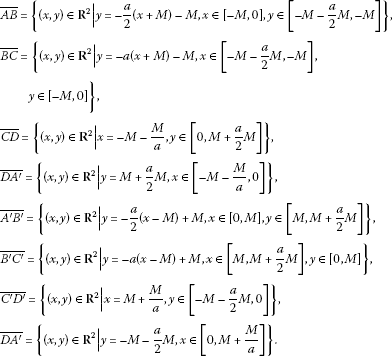Let $\mathrm{\Xi }:=\left\{\left(x,y,t\right)|\left(x,y\right)\in D,t\in \mathbf{R}\right\}$. For every ${t}_{0}\in \mathbf{R}$, $\left({x}_{0},{y}_{0}\right)\in \mathrm{\Gamma }$, we claim that ${q}_{k}\left(t,{t}_{0},{x}_{0},{y}_{0}\right)\in \mathrm{\Xi }$ for all $t>{t}_{0}$. We only need to prove this claim for every solution starting with the cylindrical surface $\mathrm{\Gamma }×\mathbf{R}$.

If $\left({x}_{0},{y}_{0}\right)\in \overline{AB}$, by (H2), we have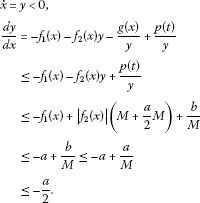Then the curve cannot leave Ξ from $\overline{AB}×\mathbf{R}$ for $t>{t}_{0}$.

If $\left({x}_{0},{y}_{0}\right)\in \overline{BC}$, since $x\le M$, by (H2), we have $|g\left(x\right)|\ge b$. Then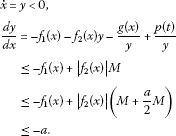Then the curve cannot leave Ξ from $\overline{BC}×\mathbf{R}$ for $t>{t}_{0}$.

If $\left({x}_{0},{y}_{0}\right)\in \overline{CD}$, from $\stackrel{˙}{x}=y>0$, so the curve cannot leave Ξ from $\overline{CD}×\mathbf{R}$ for $t>{t}_{0}$.

If $\left({x}_{0},{y}_{0}\right)\in \overline{D{A}^{\prime }}$, by (H2),(H3), we have

$\begin{array}{rcl}\stackrel{˙}{y}& =& -{f}_{1}\left(x\right)y-{f}_{2}\left(x\right){y}^{2}-g\left(x\right)+p\left(t\right)\\ =& \left(M+\frac{a}{2}M\right)\left[-{f}_{1}\left(x\right)-{f}_{2}\left(x\right)\left(M+\frac{a}{2}M\right)\right]-g\left(x\right)+p\left(t\right)\\ \le & \left(M+\frac{a}{2}M\right)\left[-{f}_{1}\left(x\right)+|{f}_{2}\left(x\right)|\left(M+\frac{a}{2}M\right)\right]-g\left(x\right)+p\left(t\right)\\ \le & -a\left(M+\frac{a}{2}M\right)-g\left(-M-\frac{M}{a}\right)+a\\ \le & -a\left(M+\frac{a}{2}M\right)+\frac{a}{M}\left(M+\frac{M}{a}\right)+a\\ \le & -a\left(M+\frac{a}{2}M\right)+2a+1\\ \le & -a\left(M-2\right)-\frac{{a}^{2}M}{2}+1\\ \le & 0.\end{array}$

Then the curve cannot leave Ξ from $\overline{D{A}^{\prime }}×\mathbf{R}$ for $t>{t}_{0}$.

Since the region D is symmetrical, we can prove that the curve cannot leave Ξ from $\overline{{A}^{\prime }{B}^{\prime }}\cup \overline{{B}^{\prime }{C}^{\prime }}\cup \overline{{C}^{\prime }{D}^{\prime }}\cup \overline{{D}^{\prime }A}×\mathbf{R}$ for $t>{t}_{0}$. Then we complete the proof of the claim and this lemma. □

From the combination of Lemma 2.2 and the Massera’s theorem (see ), we have the following lemma.

Lemma 2.2 Suppose that (H1)-(H3) hold, and p is a T-periodic function. Then (4) has a T-periodic solution$x\left(t\right)$satisfying$|x\left(t\right)|+|\stackrel{˙}{x}\left(t\right)|\le B$, where B depends on D.

Remark 2.3 From the construction of D, we can take $\left(2+\frac{1}{a}+\frac{a}{2}\right)M$ as a value of B in Lemma 2.2.

Now we consider the following two periodic equations

$\stackrel{¨}{x}+{f}_{1}\left(x\right)\stackrel{˙}{x}+{f}_{2}\left(x\right){\stackrel{˙}{x}}^{2}+g\left(x\right)=p\left(t\right),$
(7)

and

$\stackrel{¨}{x}+{f}_{1}\left(x\right)\stackrel{˙}{x}+{f}_{2}\left(x\right){\stackrel{˙}{x}}^{2}+g\left(x\right)=\varphi \left(t\right),$
(8)

where p is ${\omega }_{1}$-periodic function and ϕ is ${\omega }_{2}$-periodic function. Suppose that all conditions in Theorem 2.1 hold and

$\underset{t\in \mathbf{R}}{sup}|p\left(t\right)|\le \underset{t\in \mathbf{R}}{sup}|\varphi \left(t\right)|<+\mathrm{\infty }.$

Then, we obtain two periodic solutions $\left({x}_{1}\left(t\right),{\stackrel{˙}{x}}_{1}\left(t\right)\right)$ of (7) and $\left({x}_{2}\left(t\right),{\stackrel{˙}{x}}_{2}\left(t\right)\right)$ of (8), from the construction of D in Theorem 2.1, we can obtain two Jordan domains ${D}_{p}$ of (7) and ${D}_{\varphi }$ of (8) such that ${D}_{p}\subset {D}_{\varphi }$. The trajectory of $\left({x}_{1}\left(t\right),{\stackrel{˙}{x}}_{1}\left(t\right)\right)$ is contained in ${D}_{p}$ and the trajectory of $\left({x}_{2}\left(t\right),{\stackrel{˙}{x}}_{2}\left(t\right)\right)$ is contained in ${D}_{\varphi }$. Hence, we can find a region ${D}_{0}$ which is independent of ${D}_{p}$ and contains both the two trajectories.

For each $k\in \mathbf{N}$, let ${p}_{k}:\mathbf{R}\to \mathbf{R}$ be a 2k-periodic function such that ${p}_{k}\left(t\right)=p\left(t\right)$ for all $t\in \left[-k,k\right]$. Since $p\left(-k\right)=p\left(k\right)$ as indicated in (H1), we see that ${p}_{k}$ is continuous on the whole R. Now, we consider a series of periodic equations

$\stackrel{¨}{x}+{f}_{1}\left(x\right)\stackrel{˙}{x}+{f}_{2}\left(x\right){\stackrel{˙}{x}}^{2}+g\left(x\right)={p}_{k}\left(t\right),$

or periodic systems

$\begin{array}{r}\stackrel{˙}{x}=y,\\ \stackrel{˙}{y}=-\left[{f}_{1}\left(x\right)+{f}_{2}\left(x\right)y\right]y-g\left(x\right)+{p}_{k}\left(t\right).\end{array}$
(9)

Noting that ${sup}_{t\in \mathbf{R}}|{p}_{k}\left(t\right)|\le {sup}_{t\in \mathbf{R}}|p\left(t\right)|<+\mathrm{\infty }$ for every $k\in \mathbf{N}$, and from the discussion above we can get results as follows.

Lemma 2.4 Suppose that conditions (H1)-(H3) hold. Then system (9) possesses a 2k-periodic solution${q}_{k}$for every$k\in \mathbf{N}$. Moreover, there exists a constant B independent of k such that$|{q}_{k}|:={max}_{t\in \left[-k,k\right]}\left\{|{x}_{k}\left(t\right)|,|{y}_{k}\left(t\right)|\right\}\le B$.

Now, for the sequence of periodic solutions ${\left\{{q}_{k}\right\}}_{k\in \mathbf{N}}$ determined in Lemma 2.4, we obtain the following lemma by using the Ascoli-Arzela theorem.

Lemma 2.5 There exist a subsequence${\left\{{q}_{i}\right\}}_{i\in \mathbf{N}}$of${\left\{{q}_{k}\right\}}_{k\in \mathbf{N}}$and continuous functions${q}_{0}:\mathbf{R}\to {\mathbf{R}}^{2}$and${\stackrel{˜}{q}}_{0}:\mathbf{R}\to {\mathbf{R}}^{2}$such that${q}_{i}\to {q}_{0}$, ${\stackrel{˙}{q}}_{i}\to {\stackrel{˜}{q}}_{0}$, as$i\to +\mathrm{\infty }$, in${C}_{b}\left(\mathbf{R},{\mathbf{R}}^{2}\right)$.

Proof For each $t,{t}_{0}\in \mathbf{R}$, $t\ge {t}_{0}$, by Lemma 2.4, there exists a constant ${B}_{1}>0$ such that $|-{f}_{1}\left({x}_{k}\left(s\right)\right){y}_{k}\left(s\right)-{f}_{2}\left(x\left(s\right)\right){y}_{k}^{2}\left(s\right)-g\left({x}_{k}\left(s\right)\right)|\le {B}_{1}$. We note that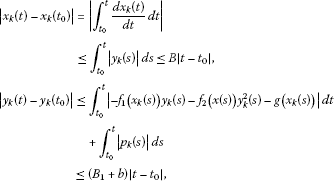and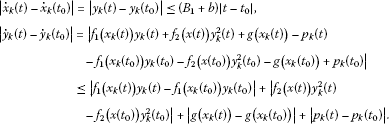For $|{\stackrel{˙}{y}}_{k}\left(t\right)-{\stackrel{˙}{y}}_{k}\left({t}_{0}\right)|$, since ${x}_{k}\left(t\right)$ is bounded, we can define

$max|f\left({x}_{k}\left(t\right)\right)|:={r}_{1}\ge 0.$

By the conditions (H2), we can get the estimation as follows.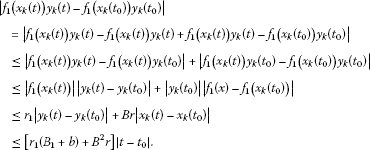Let

$max|{f}_{2}\left({x}_{k}\left(t\right)\right)\left({y}_{k}\left(t\right)+{y}_{k}\left({t}_{0}\right)\right)|:={r}_{2}\ge 0,$

we have

$\begin{array}{c}|{f}_{2}\left({x}_{k}\left(t\right)\right){y}_{k}^{2}\left(t\right)-{f}_{2}\left({x}_{k}\left({t}_{0}\right)\right){y}_{k}^{2}\left({t}_{0}\right)|\hfill \\ \phantom{\rule{1em}{0ex}}=|{f}_{2}\left({x}_{k}\left(t\right)\right){y}_{k}^{2}\left(t\right)-{f}_{2}\left({x}_{k}\left(t\right)\right){y}_{k}^{2}\left(t\right)+{f}_{2}\left({x}_{k}\left(t\right)\right){y}_{k}^{2}\left(t\right)-{f}_{2}\left({x}_{k}\left({t}_{0}\right)\right){y}_{k}^{2}\left({t}_{0}\right)|\hfill \\ \phantom{\rule{1em}{0ex}}\le |{f}_{2}\left({x}_{k}\left(t\right)\right){y}_{k}^{2}\left(t\right)-{f}_{2}\left({x}_{k}\left(t\right)\right){y}_{k}^{2}\left({t}_{0}\right)|+|{f}_{2}\left({x}_{k}\left(t\right)\right){y}_{k}^{2}\left({t}_{0}\right)-{f}_{2}\left({x}_{k}\left({t}_{0}\right)\right){y}_{k}^{2}\left({t}_{0}\right)|\hfill \\ \phantom{\rule{1em}{0ex}}\le |{f}_{2}\left({x}_{k}\left(t\right)\right)\left({y}_{k}\left(t\right)+{y}_{k}\left({t}_{0}\right)\right)||{y}_{k}\left(t\right)-{y}_{k}\left({t}_{0}\right)|+|{y}_{k}^{2}\left({t}_{0}\right)||{f}_{2}\left(x\right)-{f}_{2}\left({x}_{k}\left({t}_{0}\right)\right)|\hfill \\ \phantom{\rule{1em}{0ex}}\le {r}_{2}|{y}_{k}\left(t\right)-{y}_{k}\left({t}_{0}\right)|+{B}^{2}r|{x}_{k}\left(t\right)-{x}_{k}\left({t}_{0}\right)|\hfill \\ \phantom{\rule{1em}{0ex}}\le \left[{r}_{2}\left({B}_{1}+b\right)+{B}^{3}r\right]|t-{t}_{0}|.\hfill \end{array}$

Moreover, by (H3), we have

$|g\left({x}_{k}\left(t\right)\right)-g\left({x}_{k}\left({t}_{0}\right)\right)|\le \frac{a}{M}|{x}_{k}\left(t\right)-{x}_{k}\left({t}_{0}\right)|\le B\frac{a}{M}|t-{t}_{0}|.$

Since p is continuous and bounded, there is a constant ${r}_{3}>0$ such that

$|{p}_{k}\left(t\right)-{p}_{k}\left({t}_{0}\right)|\le {r}_{3}|t-{t}_{0}|.$

Hence, we obtain

$|{\stackrel{˙}{y}}_{k}\left(t\right)-{\stackrel{˙}{y}}_{k}\left({t}_{0}\right)|\le \left\{\left[{r}_{1}\left({B}_{1}+b\right)+{B}^{2}r\right]+\left[{r}_{2}\left({B}_{1}+b\right)+{B}^{3}r\right]+B\frac{a}{M}+{r}_{3}\right\}|t-{t}_{0}|.$

So ${\left\{{q}_{k}\right\}}_{k\in \mathbf{N}}$ and ${\left\{{\stackrel{˙}{q}}_{k}\right\}}_{k\in \mathbf{N}}$ are both equicontinuous. On the other hand, from Lemma 2.4, ${\left\{{q}_{k}\right\}}_{k\in \mathbf{N}}$ is bounded uniformly, and so does ${\left\{{\stackrel{˙}{q}}_{k}\right\}}_{k\in \mathbf{N}}$. Hence we obtain the existence of a subsequence ${\left\{{q}_{i}\right\}}_{i\in \mathbf{N}}$ convergent to a certain ${q}_{0}:=\left({x}_{0}\left(t\right),{y}_{0}\left(t\right)\right)$ in ${C}_{b}\left(\mathbf{R},{\mathbf{R}}^{2}\right)$ by Ascoli-Arzela theorem. □

Remark 2.6 We cannot conclude that the convergence of the subsequence ${\left\{{q}_{i}\right\}}_{i\in \mathbf{N}}$ is uniform for $t\in \mathbf{R}$. However, for every $a,b\in \mathbf{R}$, $a, the uniform convergence holds on $\left[a,b\right]$.

To be convenient, we still represent ${\left\{{q}_{i}\right\}}_{i\in \mathbf{N}}$ by $\left\{{q}_{k}\right\}$. For each $k\in \mathbf{N}$, let ${E}_{2k}\subset {C}_{b}\left(\mathbf{R},\mathbf{R}\right)$, denote the Banach space of continuous 2k-periodic functions on R with values in R under the norm

${\parallel x\parallel }_{{E}_{k}}={\left({\int }_{-k}^{k}{|x\left(t\right)|}^{2}\phantom{\rule{0.2em}{0ex}}dt\right)}^{\frac{1}{2}}.$

Now, we prove the main result that ${q}_{0}$ is the desired homoclinic solution of (5).

Lemma 2.7 The function${q}_{0}$determined by Lemma 2.5 is a nontrivial homoclinic solution of (5).

Proof The proof will be divided into three steps.

Step 1. We show that ${q}_{0}$ is a solution of (9). For every $k\in \mathbf{N}$ and $t\in \mathbf{R}$ we have

$\begin{array}{r}\stackrel{˙}{{x}_{k}}={y}_{k},\\ \stackrel{˙}{{y}_{k}}=-\left[{f}_{1}\left({x}_{k}\right)+{f}_{2}\left({x}_{k}\right){y}_{k}\right]{y}_{k}-g\left({x}_{k}\right)+{p}_{k}\left(t\right).\end{array}$
(10)

For each fixed $a,b\in \mathbf{R}$, $a, since ${q}_{k}\to {q}_{0}$ and ${p}_{k}\to p$ on $\left[a,b\right]$ uniformly, we have

$\begin{array}{rl}{\stackrel{˙}{q}}_{k}=& \left(\frac{d{x}_{k}\left(t\right)}{dt},\frac{d{y}_{k}\left(t\right)}{dt}\right)\to w\\ :=& \left({y}_{0}\left(t\right),-\left[{f}_{1}\left({x}_{0}\left(t\right)\right)+{f}_{2}\left({x}_{0}\left(t\right)\right){y}_{0}\left(t\right)\right]{y}_{0}\left(t\right)-g\left({x}_{0}\left(t\right)\right)+p\left(t\right)\right)\end{array}$
(11)

on $\left[a,b\right]$ uniformly. Hence there exists a constant ${k}_{0}\in \mathbf{N}$ such that (10) can be transformed into

$\begin{array}{c}\stackrel{˙}{{x}_{k}}={y}_{k},\hfill \\ \stackrel{˙}{{y}_{k}}=-\left[{f}_{1}\left({x}_{k}\right)+{f}_{2}\left({x}_{k}\right){y}_{k}\right]{y}_{k}-g\left({x}_{k}\right)+p\left(t\right)\hfill \end{array}$

for each $k\ge {k}_{0}$ and $t\in \left[a,b\right]$. So ${\stackrel{˙}{q}}_{k}$ is continuous on $\left[a,b\right]$ for $k\ge {k}_{0}$. Note the fact that ${\stackrel{˙}{q}}_{k}$ is a derivative of ${q}_{k}$ in $\left[a,b\right]$ for every $k\ge {k}_{0}$ and ${\stackrel{˙}{q}}_{k}$ converges to ${\stackrel{˜}{q}}_{0}$ uniformly in $\left[a,b\right]$ by Lemma 2.5. Since (11) holds and ${q}_{k}\to {q}_{0}$ uniformly on $\left[a,b\right]$, we have $w={\stackrel{˙}{q}}_{0}$ in $\left(a,b\right)$. Because a and b are arbitrary, we conclude that $w={\stackrel{˙}{q}}_{0}$ in R and ${q}_{0}$ satisfies (5). Moreover, we have actually proved that $\left\{{q}_{k}\right\}$ converges to ${q}_{0}$ in ${C}_{b}\left(\mathbf{R},{\mathbf{R}}^{2}\right)$.

Step 2. We prove that ${x}_{0}\left(t\right)\to 0$, as $t\to ±\mathrm{\infty }$. We note that

$\stackrel{˙}{{x}_{k}}\stackrel{¨}{{x}_{k}}=-g\left({x}_{k}\right)\stackrel{˙}{{x}_{k}}-{f}_{1}\left({x}_{k}\right){\left(\stackrel{˙}{{x}_{k}}\right)}^{2}-{f}_{2}\left({x}_{k}\right){\left(\stackrel{˙}{{x}_{k}}\right)}^{3}+{p}_{k}\left(t\right)\stackrel{˙}{{x}_{k}},$
(12)

by Lemmas 2.1 and 2.2, we have

${\stackrel{˙}{x}}_{k}\le M+\frac{aM}{2},$

from (H2), we obtain

${f}_{1}\left({x}_{k}\right)+{f}_{2}\left({x}_{k}\right)\stackrel{˙}{{x}_{k}}\ge {f}_{1}\left({x}_{k}\right)-|{f}_{2}\left({x}_{k}\right)|\left(M+\frac{aM}{2}\right)\ge a.$

Integrating (12) from −k to k, we have

${\int }_{-k}^{k}\left({f}_{1}\left({x}_{k}\right)+{f}_{2}\left({x}_{k}\right)\left(\stackrel{˙}{{x}_{k}}\right)\right){\left(\stackrel{˙}{{x}_{k}}\right)}^{2}\phantom{\rule{0.2em}{0ex}}dt={\int }_{-k}^{k}{p}_{k}\left(t\right)\stackrel{˙}{{x}_{k}}\phantom{\rule{0.2em}{0ex}}dt.$

So

$\begin{array}{rcl}a{\parallel \stackrel{˙}{{x}_{k}}\parallel }_{{E}_{k}}^{2}& \le & |{\int }_{-k}^{k}\left({f}_{1}\left({x}_{k}\right)+{f}_{2}\left({x}_{k}\right)\left(\stackrel{˙}{{x}_{k}}\right)\right){\left(\stackrel{˙}{{x}_{k}}\right)}^{2}\phantom{\rule{0.2em}{0ex}}dt|\\ \le & {\parallel {p}_{k}\parallel }_{{E}_{k}}{\parallel \stackrel{˙}{{x}_{k}}\parallel }_{{E}_{k}}.\end{array}$

Hence, by (H1), there is a constant ${d}_{1}>0$ independent of k such that

${\parallel \stackrel{˙}{{x}_{k}}\parallel }_{{E}_{k}}={\parallel {y}_{k}\parallel }_{{E}_{k}}\le {d}_{1}.$
(13)

On the other hand, we have

${x}_{k}\stackrel{¨}{{x}_{k}}=-g\left({x}_{k}\right){x}_{k}-{f}_{1}\left({x}_{k}\right)\stackrel{˙}{{x}_{k}}{x}_{k}-{f}_{2}\left({x}_{k}\right){\left(\stackrel{˙}{{x}_{k}}\right)}^{2}{x}_{k}+{p}_{k}\left(t\right){x}_{k}.$
(14)

From Lemmas 2.1 and 2.2, there exists a constant ${m}_{1}>0$ independent of k such that

$|{f}_{2}\left({x}_{k}\right){\left(\stackrel{˙}{{x}_{k}}\right)}^{2}|\le {m}_{1}.$

Let ${m}_{2}={min}_{\left(x,\stackrel{˙}{x}\right)\in D}{g}^{\prime }\left(x\right)$, since ${g}^{\prime }\left(x\right)>0$, we have ${m}_{2}>0$ and $|g\left({x}_{k}\right)|\ge {m}_{2}|{x}_{k}|$. Integrating (14) from −k to k, we have

${d}_{1}^{2}={\parallel \stackrel{˙}{{x}_{k}}\parallel }_{{E}_{k}}^{2}={\int }_{-k}^{k}{\left(\stackrel{˙}{{x}_{k}}\right)}^{2}\phantom{\rule{0.2em}{0ex}}dt\ge {m}_{2}{\parallel {x}_{k}\parallel }_{{E}_{k}}^{2}-\left({m}_{1}+b\right)\parallel {x}_{k}\parallel .$

Then there exists a constant ${d}_{2}>0$ independent of k such that

${\parallel {x}_{k}\parallel }_{{E}_{k}}\le {d}_{2}.$
(15)

We note that

${\parallel {x}_{0}\parallel }_{{L}^{2}}^{2}=\underset{i\to +\mathrm{\infty }}{lim}{\int }_{-i}^{i}{|{x}_{0}\left(t\right)|}^{2}\phantom{\rule{0.2em}{0ex}}dt=\underset{i\to +\mathrm{\infty }}{lim}\underset{k\to +\mathrm{\infty }}{lim}{\int }_{-i}^{i}{|{x}_{k}\left(t\right)|}^{2}\phantom{\rule{0.2em}{0ex}}dt.$

Obviously,

${\int }_{-i}^{i}{|{x}_{k}\left(t\right)|}^{2}\phantom{\rule{0.2em}{0ex}}dt\le {\int }_{-k}^{k}{|{x}_{k}\left(t\right)|}^{2}\phantom{\rule{0.2em}{0ex}}dt={\parallel {x}_{k}\parallel }_{{E}_{k}}^{2}\le {d}_{2}^{2}$

holds for every $i\in \mathbf{N}$ and $k\in \mathbf{N}$, $k\ge i$. Since ${x}_{k}\to {x}_{0}$ on $\left[-i,i\right]$ uniformly. Let $k\to +\mathrm{\infty }$, we obtain

${\int }_{-i}^{i}{|{x}_{0}\left(t\right)|}^{2}\phantom{\rule{0.2em}{0ex}}dt\le {d}_{2}^{2},$

and let $i\to +\mathrm{\infty }$, we have

${\int }_{-\mathrm{\infty }}^{+\mathrm{\infty }}{|{x}_{0}\left(t\right)|}^{2}\phantom{\rule{0.2em}{0ex}}dt\le {d}_{2}^{2}.$

Hence,

$\underset{r\to \mathrm{\infty }}{lim}{\int }_{|t|\ge r}{|{x}_{0}\left(t\right)|}^{2}\phantom{\rule{0.2em}{0ex}}dt=0.$
(16)

This implies that ${x}_{0}\left(t\right)\to 0$ as $t\to ±\mathrm{\infty }$. Similarly, ${y}_{0}\left(t\right)\to 0$ as $t\to ±\mathrm{\infty }$.

Step 3. $p\left(t\right)\not\equiv 0$ implies that ${q}_{0}$ is nontrivial.

We complete this lemma. □

Finally, Theorem 1.1 is proved by summarizing the results in Lemmas 2.1 and 2.7.

## References

1. Buica A, Gasull A, Yang J: The third order Melnikov function of a quadratic center under quadratic perturbations. J. Math. Anal. Appl. 2007, 331: 443–454. 10.1016/j.jmaa.2006.09.008

2. Champneys A, Lord G: Computation of homoclinic solutions to periodic orbits in a reduced water-wave problem. Physica D, Nonlinear Phenom. 1997, 102: 101–124. 10.1016/S0167-2789(96)00206-0

3. Chow S, Hale J, Mallet-Paret J: An example of bifurcation to homoclinic orbits. J. Differ. Equ. 1980, 37: 351–371. 10.1016/0022-0396(80)90104-7

4. Li C, Rousseau C: A system with three limit cycles appearing in a Hopf bifurcation and dying in a homoclinic bifurcation: the cusp of order 4. J. Differ. Equ. 1989, 79: 132–167. 10.1016/0022-0396(89)90117-4

5. Zhu C, Zhang W: Computation of bifurcation manifolds of linearly independent homoclinic orbits. J. Differ. Equ. 2008, 245: 1975–1994. 10.1016/j.jde.2008.06.029

6. Andronov A, Vitt E, Khaiken S: Teory of Oscillators. Pergamon, Oxford; 1966.

7. Guckenheimer J, Holmes P: Nonlinear Oscillation, Dynamical Systems, and Bifurcations of Vector Fields. Springer, New York; 1983.

8. Rabinowitz P: Homoclinic orbits for a class of Hamiltonian systems. Proc. R. Soc. Edinb. A 1990, 114: 33–38. 10.1017/S0308210500024240

9. Ambrosetti A, Rabinowitz P: Dual variational methods in critical point theory and applications. J. Funct. Anal. 1973, 14: 349–381. 10.1016/0022-1236(73)90051-7

10. Chow S, Hale J: Methods of Bifurcation Theory. Springer, New York; 1982.

11. Carrião P, Miyagaki O: Existence of homoclinic solutions for a class of time-dependent Hamiltonian systems. J. Math. Anal. Appl. 1999, 230: 157–172. 10.1006/jmaa.1998.6184

12. Szulkin A, Zou W: Homoclinic orbits for asymptotically linear Hamiltonian systems. J. Funct. Anal. 2001, 187: 25–41. 10.1006/jfan.2001.3798

13. Izydorek M, Janczewska J: Homoclinic solutions for a class of the second order Hamiltonian systems. J. Differ. Equ. 2005, 219: 375–389. 10.1016/j.jde.2005.06.029

14. Izydorek M, Janczewska J: Homoclinic solutions for nonautonomous second order Hamiltonian systems with a coercive potential. J. Math. Anal. Appl. 2007, 335: 1119–1127. 10.1016/j.jmaa.2007.02.038

15. Tang X, Xiao L: Homoclinic solutions for nonautonomous second-order Hamiltonian systems with a coercive potential. J. Math. Anal. Appl. 2009, 351: 586–594. 10.1016/j.jmaa.2008.10.038

16. Tang X, Xiao L: Homoclinic solutions for a class of second-order Hamiltonian systems. J. Math. Anal. Appl. 2009, 354: 539–549. 10.1016/j.jmaa.2008.12.052

17. Zhang Y: Homoclinic solutions for a forced Liénard type system. Results Math. 2010, 57: 69–78. 10.1007/s00025-009-0005-9

18. Mawhin J, Ward J: Periodic solutions of second order forced Liénard differential equations at resonance. Arch. Math. 1983, 41: 337–351. 10.1007/BF01371406

19. Omari P, Villari G, Zanolin F: Periodic solutions of Liénard differential equations with one-sided growth restriction. J. Differ. Equ. 1987, 67: 278–293. 10.1016/0022-0396(87)90151-3

20. Franks J: Generalizations of the Poincaré-Birkhoff theorem. Ann. Math. 1988, 128: 139–151. 10.2307/1971464

21. Jacobowitz H:Periodic solutions of $\stackrel{¨}{x}+f\left(x,t\right)=0$ via Poincaré-Birkhoff theorem. J. Differ. Equ. 1976, 20: 37–52. 10.1016/0022-0396(76)90094-2

22. Zhang Y: Eventually vanished solutions of a forced Liénard system. Appl. Math. Mech. 2009, 30(10):1335–1344. 10.1007/s10483-009-1013-6

23. Rao MRM: Ordinary Differential Equations. Affiliated East-West Private Limited, London; 1980.

24. Antosiewicz H: On nonlinear differential equations of the second order with integrable forcing term. J. Lond. Math. Soc. 1955, 30: 64–67. 10.1112/jlms/s1-30.1.64

25. Atslega S, Sadyrbaev F: Multiple period annuli in Liénard type equations. Appl. Math. Lett. 2010, 23(2):165–169. 10.1016/j.aml.2009.09.006

26. Chang S, Chang H: Existence of periodic solutions of nonlinear systems of a generalized Liénard type. Kyungpook Math. J. 1999, 39(2):351–365.

27. Esmailzadeh E, Mehri B, Nakhaie-Jazar G: Periodic solution of a second order, autonomous, nonlinear system. Nonlinear Dyn. 1996, 10(4):307–316. 10.1007/BF00045479

28. Feng Z: Exact solutions to the Liénard equation and its applications. Chaos Solitons Fractals 2004, 21: 343–348. 10.1016/j.chaos.2003.10.025

29. Qian C: Boundedness and asymptotic behavior of solutions of second order nonlinear system. Bull. Lond. Math. Soc. 1992, 24: 281–288. 10.1112/blms/24.3.281

30. Sansone G, Conti R: Nonlinear Differential Equations. Pergamon, New York; 1964.

31. Hesaaraki M, Moradifam A: Intersection with the vertical isocline in the generalized Liénard equations. J. Math. Anal. Appl. 2007, 334(2):787–798. 10.1016/j.jmaa.2007.01.015

32. Zhou Q, Shao J, Zhou J: Existence and uniqueness of periodic solutions for a class of generalized Liénard systems with forcing term. J. Comput. Appl. Math. 2009, 224: 743–750. 10.1016/j.cam.2008.06.006

33. Massera JL: On Liapunoff’s conditions of stability. Ann. Math. 1949, 50: 705–721. 10.2307/1969558

## Acknowledgements

This work was supported by the Sichuan Provincial Department of Education Fund (12ZA068), and the projects of Leshan Normal University (Z1164, Z1006).

## Author information

Authors

### Corresponding author

Correspondence to Yongxin Zhang.

### Competing interests

The author declares that he has no competing interests.

## Authors’ original submitted files for images

Below are the links to the authors’ original submitted files for images.

## Rights and permissions

Reprints and Permissions

Zhang, Y. Homoclinic solutions for a forced generalized Liénard system. Adv Differ Equ 2012, 94 (2012). https://doi.org/10.1186/1687-1847-2012-94

• Accepted:

• Published:

• DOI: https://doi.org/10.1186/1687-1847-2012-94

### Keywords

• homoclinic
• bounded solution
• non-Hamiltonian
• accumulation